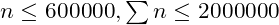# [NOI2018]冒泡排序0 0 0 0 0 i 0 0 1 1 0 0 1 0

emmmmm大概就是说一共会有n-p[i]个1，p[i]-1个0。而且很显然，包含这个i的逆序对，仅仅是以i为左端点的就有右面的0那么多，就是p[i]-i那么多。如果i左边有1，右面的零就会更多，左边的1也可以和i形成逆序对，那么就会超出限制。这样我们就知道对于这个i所有左边的元素都小于它。再同理讨论p[i]<i和p[i]=i的情况，我们发现由于没有元素可以当下降子序列的中间的元素，显然是没有长度为3的下降子序列的。

（上面两段段来自武弘勋的知乎回答

seq = ""
mx = 0
for i in range(1,n+1):
if p[i]>mx:
seq+="("*(p[i]-mx)
mx = p[i]
seq+=")"

dbq，12点30分了，我不能写了，我好爬啊，希望我能做更多更好的题。

#include <bits/stdc++.h>

using namespace std;
typedef long long ll;
#define set0(x) memset(x,0,sizeof(x))
#define F first
#define S second
#define PB push_back
#define MP make_pair
#define rep(i, a, b) for(int i = a; i < (b); ++i)
#define trav(a, x) for(auto& a : x)
#define all(x) x.begin(), x.end()
#define sz(x) (int)(x).size()

ll chkmax(ll &a,ll b){return a = a>b?a:b;}
typedef long long ll;
typedef pair<int,int> pii;
typedef pair<ll,ll> pll;
typedef vector<int> VI;
x = 0;char ch = getchar();ll f = 1;
while(!isdigit(ch)){if(ch == '-')f*=-1;ch=getchar();}
while(isdigit(ch)){x = x*10+ch-48;ch=getchar();}x*=f;
}
template<typename T, typename... Args> void read(T &first, Args& ... args) {
}
int mod = 998244353;
inline int mul(int x,int y){return 1ll*x*y%mod;}
inline int add(int x,int y){return x+y>=mod?x+y-mod:x+y;}
inline int sub(int x,int y){return x-y<0?x-y+mod:x-y;}
inline int sq(int x){return 1ll*x*x%mod;}
int mpow(int a,int b){return b == 0 ? 1 : ( b&1 ? mul(a,sq(mpow(a,b/2))) : sq(mpow(a,b/2)));}
const int N = 1200000;
int fac[N+10],invfac[N+10];
int C(int n,int m){
if(n<0 || m<0 || m>n)return 0;
return mul(fac[n],mul(invfac[m],invfac[n-m]));
}
int C1(int n,int m){
return sub(C(n,m+(n-m)/2),C(n,m+2+(n-m-2)/2));
}
int T,n;

void solve(){
vector<int> a(n+1),vis(n+2,0);
int mx = 0,mi = 0,cx = 0,cy = 0,ans = 0;
for(int i=1;i<=n;i++){
while(vis[mi+1])mi+=1;
vis[a[i]] = 1;
if(a[i]<mx && a[i]>mi+1){
break;
}
if(a[i] == mi+1 && a[i]<mx){
cx+=1;
cy-=1;
}else{
int d = a[i]-mx;
cx+=d+1;
cy+=d-1;
}
mx = max(mx,a[i]);
}
cout<<ans<<endl;
}

int main(){
fac = 1;
for(int i=1;i<=N;i++)fac[i] = mul(fac[i-1],i);
invfac[N] = mpow(fac[N],mod-2);
for(int i=N-1;i>=0;i--) invfac[i] = mul(invfac[i+1],i+1);